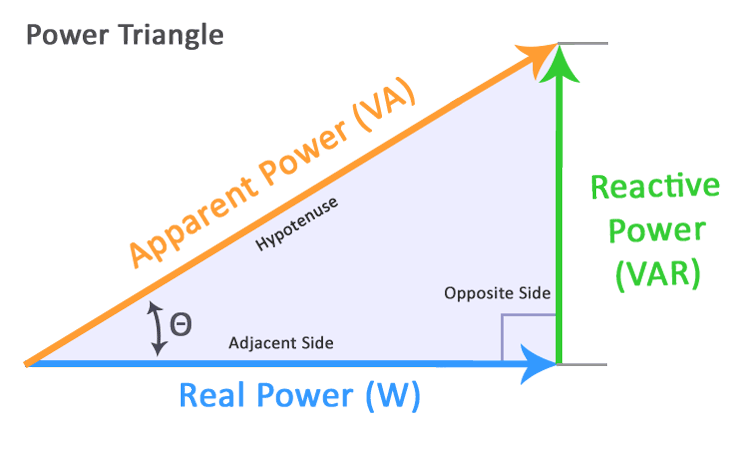##### Power Triangle Calculator
Choose the values you want to find, then enter the known quantities and press Calculate.

##### Power Triangle##### Terms
• Real Power (P) - Measured in watts, defines the power consumed by the resistive part of a circuit. Also known as true or active power, performs the “real work” within an electrical circuit.
• Reactive Power (Q) - Measured in VAR, the power consumed in an AC circuit that does not perform any useful work, caused by inductors and capacitors. Reactive power counteracts the effects of real power, taking power away from a circuit for use in magnetic fields.
• Apparent Power (S) - The product of RMS voltage and rms current flowing into a circuit, contains real power and reactive power.
• Power Factor (θ) - The ratio of real power (P) to apparent power (S), generally expressed as either a decimal or percentage value. Power factor defines the phase angle between the current and voltage waveforms. The larger the phase angle, the greater the reactive power.
##### Formulas
• Real Power (P) = VIcos θ, Watts (W)
• Reactive Power (Q) = VIsin θ, Volt-amperes Reactive (VAr)
• Apparent Power (S) = VI, Volt-amperes (VA)
• Power Factor (θ) = P/S
• VA = W / cos θ
• VA = VAR / sin θ
• VAR = VA * sin θ
• VAR = W * tan θ
• W = VA * cos θ
• W = VAR / tan θ
• Sin(θ) = Opposite / Hypotenuse = Q/S = VAr/VA
• Cos(θ) = Adjacent / Hypotenuse = P/S = W/VA = power factor, p.f.
• Tan(θ) = Opposite / Adjacent = Q/P = VAr/W

🌐 Connect with like-minded professionals in the electrical testing industry. Our knowledge sharing hub offers a supportive community where you can exchange insights, learn from real-world experiences, and stay at the forefront of electrical testing practices. Join us and expand your expertise!